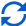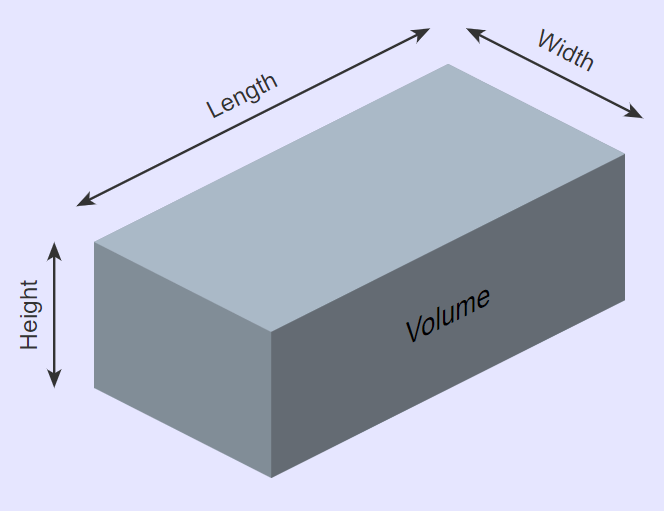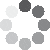Enter parameters
Enter parameters
LENGTH, WIDTH & DEPTH
Length:
ft
• inches (in)
• feet (ft)
• yards (yd)
• centimeters (cm)
• meters (m)
Width:
ft
• inches (in)
• feet (ft)
• yards (yd)
• centimeters (cm)
• meters (m)
Height:
ft
• inches (in)
• feet (ft)
• yards (yd)
• centimeters (cm)
• meters (m)
Price per unit of volume:
ft³
• cubic yards (yd³)
• cubic feet (ft³)
• cubic inches (in³)
• cubic centimeters (cm³)
• cubic meters ()Result
Volume:
0
ft³
• cubic yards (yd³)
• cubic feet (ft³)
• cubic inches (in³)
• cubic centimeters (cm³)
• cubic meters ()
Cost:
\$(No Ratings Yet)Loading...
Similar Calculators:
Real-time graphics. Make the calculations and see the changes.
Embed
Similar Calculators:Working out the amount of space an object occupies is often necessary for a landscaping or transportation project.

It is crucial that you can calculate an accurate value, as this will save you both time and money.

We know that estimating this by eye can be very difficult and will often lead to miscalculation.

That’s why we built our online cubic feet calculator. 👍

You can use it to effortlessly calculate the volume of the shape and the associated cost as well.

In this article we will show you how effortless the calculator is to use and what it calculates.

In addition, we will detail the Mathematical formulae and equations it uses and finally end with two fully worked examples.

Contents:

## Cubic feet calculator formulaThe first step in working out the amount of space your shape occupies is to measure its length, width and depth in feet.

When you enter these measurements, our cubic feet calculator first figures out the amount of space it occupies using the equation:

$$Volume = Length \times Width \times Height$$

The standard given volume units the calculator works in is cubic footage.

Note that the top area of the shape can also be calculated using the formulae:

$$Area = Length \times Width$$

The standard units for area is square footage.

You can work out the total cost of the material using our online calculator if you know the price per unit volume for the material.

In a gardening project this might be the cost to purchase a cubic foot of the material.

If you are looking to ship material, then this might be the cost to send a cubic foot of the material by land, sea or air.

The calculator works out the total cost of your landscaping or shipping project using the mathematical formulae:

$$Cost = Price\,per\,unit\,volume \times Volume$$

## What if I don’t know the price per unit volume?

We understand that it’s unlikely you will know the cost of material or shipping per cubic feet.

However, you can work out these values yourself, by simply using the formulae:

$$Price\,per\,unit\,volume = {Total\,cost\,of\,material \over Total\,volume\,of\,aggregate}$$

## What if my measurements are not in feet?

Well, the solution couldn’t be easier! Our online calculator takes care of all the conversions for you. 🥰

You can interchangeably switch between different units – for example length in yards, width in feet and height in inches.

Simply select the units you desire to use in the drop down area and the calculator will do the following conversions for you:

$$1\,foot = 12\,inches = 0.33\,yards = 30.48\,centimeters = 0.3048\,meters$$

$$1\,US\,short\,ton = 2000\,pounds = 0.893\,imperial\,long\,ton = 907\,kg$$

Something not clear?

If you are confused, then see below for two fully worked examples.

In these we start with different initial criteria and we work out the total volume and cost of the material/shipping project.

Otherwise, enter your measurements in the calculator to make an effective and efficient start on your next landscaping project.

## Example calculations

✅ Calculate the cubic capacity working with a known price per unit volume

For our first example, let’s imagine we want to purchase some material in the shape of a cuboid.

The cuboid has dimensions 10 feet in length and 6 feet in width.

Therefore, the top area in square feet of the cuboid can be calculated by using the formulae:

$$Area = Length \times Width = 10\,ft \times 6\,ft = 60\,ft^2$$

If the height of the cuboid is 4 feet, then the calculator is able to determine the total capacity/volume of the cuboid using the formulae:

$$Volume = Length \times Width \times Height = 10\,ft \times 6\,ft \times 4\,ft = 240\,ft^3$$

Let’s say that the cost to purchase the material per cubic feet is 0.7 dollars.

Therefore, the calculator is able to figure out the total cost of purchasing the material, using the formulae:

$$Cost = Price\,per\,unit\,volume \times Volume = 0.7\,/ft^3 \times 240\,ft^3 = 168$$

✅ Shipping example calculating the price per unit volume

For our second example, let’s imagine we want to ship a box with dimensions 10 feet in length, 4 feet in width and 9 inches (0.75 feet) in height.

I don’t know the price to ship a cubic yard or foot of material, but the cost to ship 40 cubic feet of material is 60 dollars.

Using this information, I am able to calculate the price to ship material per unit of volume with the equation:

$$Price\,per\,unit\,volume = {Total\,cost\,of\,material \over Total\,volume\,of\,aggregate} = {60 \over 40\,ft^3} = 1.5\,/ft^3$$

I then enter this information into the calculator.

It firstly works out the total volume in cubic feet of the box I wish to ship:

$$Volume = Length \times Width \times Height = 10\,ft \times 4\,ft \times 0.75\,ft = 30\,ft^3$$

Then, the calculator works out the total cost to ship the box using the price per unit of volume:

$$Cost = Price\,per\,unit\,volume \times Volume = 1.5\,/ft^3 \times 30\,ft^3 = 45$$

## How to convert volume calculations into different units?

For example, you may wish to calculate cubic feet from inches.

Well, our cubic feet calculator has the option to change the volume from cubic feet to inches, yards, centimeters and meters.

The calculator uses the following conversions to find the appropriate units:

$$Volume\,in\,Cubic\,Yards\,(yd^3) = {Volume\,in\,Feet\,(ft^3) \over 27}$$

$$Volume\,in\,Cubic\,Inches\,(in^3) = Volume\,in\,Feet\,(ft^3) \times 1728$$

$$Volume\,in\,Cubic\,Meters\,(m^3) = Volume\,in\,Feet\,(ft^3) \times 0.0283$$

$$Volume\,in\,Cubic\,Centimeters\,(cm^3) = Volume\,in\,Feet\,(ft^3) \times 28,316.8$$

Embed this calculator on your site!Add live graphics
Copied to clipboard! Preview